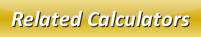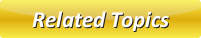Potential Energy CalculatorKinetic Energy CalculatorMechanical Energy CalculatorConservation of EnergyEnergyRenewable Sources of Energy

# Mechanical Energy Calculator

Mechanical energy can be explained as the energy which an object possess because of its position or motion. In simple words, it is the addition of kinetic and potential energy.

The Formula to calculate mechanical energy is given by:where,
M.E = Mechanical Energy [Joules]
m = Mass of an Object [kilograms]
v = Velocity [m/s]
g = Gravitation acceleration of earth [9.8m/s2]
h = Height from the ground [meters]

In our below online mechanical energy calculator, enter the input values and click calculate button to find mechanical energy.

 Mass of an Object (m): [kilograms] Velocity (v): [m/s] Height from the Ground (h): [meters] Mechanical Energy (M.E): [Joules]

Latest Calculator Release

Average Acceleration Calculator

Average acceleration is the object's change in speed for a specific given time period. ...

Free Fall Calculator

When an object falls into the ground due to planet's own gravitational force is known a...

Torque Calculator

Torque is nothing but a rotational force. In other words, the amount of force applied t...

Average Force Calculator

Average force can be explained as the amount of force exerted by the body moving at giv...

Angular Displacement Calculator

Angular displacement is the angle at which an object moves on a circular path. It is de...